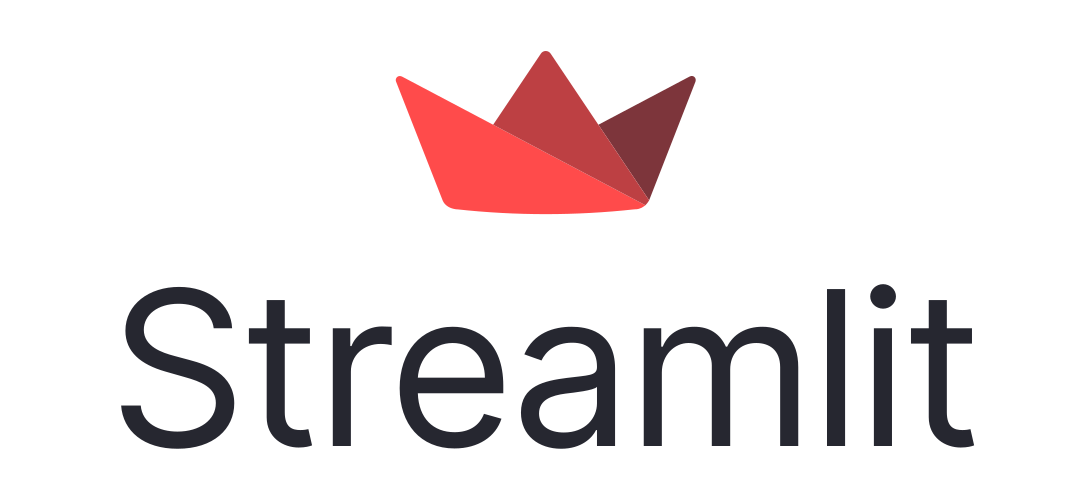# Create a Dashboard with Streamlit

Use Streamlit to create dashboards in Python and deploy them on Saturn CloudTry this example in seconds on Saturn Cloud

## Overview

With Streamlit, data scientists can produce low-code data apps by abstracting away much of the technologies and protocols typically required for interactive data visualization. Deploying a Streamlit app on Saturn Cloud gives you not only a scalable backend but also a url for dissemination.

In this example, we create a simple UI that shows a Uniform Manifold Approximation and Projection (UMAP) model projection of the famous MNIST digits and fashion datasets. The app will read the data, train the UMAP model, and produce a 3D graph of the result using plotly.

## Creating the App

All the app code is contained in a file called “app.py.” To deploy this dashboard on Saturn Cloud, call `streamlit run app.py --server.port 8000 --server.address 0.0.0.0` as the command in a Saturn Cloud deployment. It is important to set the server port and address to `"8000"` and `"0.0.0.0"` so that Saturn Cloud can correctly deploy the app. See Saturn Cloud docs for more detailed instructions on deploying this and other dashboards.

### Import the Libraries

This exercise uses Streamlit, plotly, and UMAP to create a dashboard app:

``````import numpy as np
import pandas as pd
import plotly.express as px
import streamlit as st
from umap import UMAP
``````

First, create two functions to handle data loading and processing. The first function simply reads the data from an AWS S3 bucket. The second takes that data, runs `fit_transform` on it using UMAP to create a projection, and creates a Plotly 3D scatter plot of the result.

Note that we are using `@st.cache` decorator for both functions. This decorator tells Streamlit to cache the data so that the end user doesn’t have to wait for it to download or process twice.

``````@st.cache
if selected_dataset == "MNIST-Digits":
"https://saturn-public-data.s3.us-east-2.amazonaws.com/MNIST-1000/mnist-1000-input.csv"
)
"https://saturn-public-data.s3.us-east-2.amazonaws.com/MNIST-1000/mnist-1000-labels.csv"
)
y = np.unique(y, return_inverse=True)

elif selected_dataset == "MNIST-Fashion":
"https://saturn-public-data.s3.us-east-2.amazonaws.com/MNIST-1000/fashion-1000-input.csv"
)
"https://saturn-public-data.s3.us-east-2.amazonaws.com/MNIST-1000/fashion-1000-labels.csv"
)
y = np.unique(y, return_inverse=True)

else:
X = None
y = None

return X, y

@st.cache
def create_figure(X, y):
umap_3d = UMAP(n_components=3, init="random", random_state=0)
proj_3d = umap_3d.fit_transform(X, y=y)

fig = px.scatter_3d(proj_3d, x=0, y=1, z=2, color=y)
fig.update_layout(transition_duration=500, height=1000)
fig.update(layout_coloraxis_showscale=False)
fig.update_traces(marker_size=2)

return fig
``````

### Define the App Layout and Content

After creating the functions, define the layout of the Streamlit app. Streamlit makes it easy to define text content with functions like `title`, `markdown`, and `text`. You can also define user input using functions like `selectbox` or output using functions like `plotly_chart`.

The following code displays a title with a markdown text description and creates two columns. The first column contains a dropdown selector for the dataset, and the second a chart showing the 3D graph of the UMAP projection.

``````st.set_page_config(page_title="UMAP Projections", layout="wide")

st.title("UMAP Projections for MNIST and Fashion-MNIST Datasets")

st.markdown(
"""
Uniform Manifold Approximation and Projection (UMAP) is a general-purpose dimension reduction algorithm. Similar to t-distributed stochastic neighbor embedding (t-SNE), you can use UMAP to visualize the relationships between data points. In this example, we are training a three-component UMAP model on MNIST datasets and then displaying the 3D graph of the result. The color of the point in the graph is based on the label. In the resulting graph, blobs of colors show that UMAP clustered data points with similar labels together.
"""
)

col1, col2 = st.columns([1, 3])

with col1:
selected_dataset = st.selectbox("Please select a dataset:", ("MNIST-Digits", "MNIST-Fashion"))

with col2:
fig = create_figure(X, y)
plot_3d = st.plotly_chart(create_figure(X, y), use_container_width=True)
``````

### Run the App

You then need to only load the app code to git and link the code appropriately to a Saturn Cloud deployment.

Check out our other dashboard resources for examples using other frameworks.

``````import numpy as np
import pandas as pd
import plotly.express as px
import streamlit as st
from umap import UMAP

@st.cache
if selected_dataset == "MNIST-Digits":
"https://saturn-public-data.s3.us-east-2.amazonaws.com/MNIST-1000/mnist-1000-input.csv"
)
"https://saturn-public-data.s3.us-east-2.amazonaws.com/MNIST-1000/mnist-1000-labels.csv"
)
y = np.unique(y, return_inverse=True)

elif selected_dataset == "MNIST-Fashion":
"https://saturn-public-data.s3.us-east-2.amazonaws.com/MNIST-1000/fashion-1000-input.csv"
)
"https://saturn-public-data.s3.us-east-2.amazonaws.com/MNIST-1000/fashion-1000-labels.csv"
)
y = np.unique(y, return_inverse=True)

else:
X = None
y = None

return X, y

@st.cache
def create_figure(X, y):
umap_3d = UMAP(n_components=3, init="random", random_state=0)
proj_3d = umap_3d.fit_transform(X, y=y)

fig = px.scatter_3d(proj_3d, x=0, y=1, z=2, color=y)
fig.update_layout(transition_duration=500, height=1000)
fig.update(layout_coloraxis_showscale=False)
fig.update_traces(marker_size=2)

return fig

st.set_page_config(page_title="UMAP Projections", layout="wide")

st.title("UMAP Projections for MNIST and Fashion-MNIST Datasets")

st.markdown(
"""
Uniform Manifold Approximation and Projection (UMAP) is a general-purpose dimension reduction algorithm. Similar to t-distributed stochastic neighbor embedding (t-SNE), you can use UMAP to visualize the relationships between data points. In this example, we are training a three-component UMAP model on MNIST datasets and then displaying the 3D graph of the result. The color of the point in the graph is based on the label. In the resulting graph, blobs of colors show that UMAP clustered data points with similar labels together.
"""
)

col1, col2 = st.columns([1, 3])

with col1:
selected_dataset = st.selectbox("Please select a dataset:", ("MNIST-Digits", "MNIST-Fashion"))